# Bihar Board Class 10th Maths Solutions Chapter 3 Pair of Linear Equations in Two Variables Ex 3.2

Bihar Board Class 10th Maths Solutions Chapter 3 Pair of Linear Equations in Two Variables Ex 3.2 Textbook Questions and Answers.

## BSEB Bihar Board Class 10th Maths Solutions Chapter 3 Pair of Linear Equations in Two Variables Ex 3.2

Question 1.
Form the pair of linear equations in the following problems, and find their solution graphically.

1. 10 students of Class X took part in a Mathematics quiz. If the number of girls is 4 more than the number of boys, If the number of girls is 4 more than the number of boys, find the number of boys and girls who took part in the quiz.

2. 5 pencils and 7 pens together cost Rs 50, whereas 7 pencils and 5 pens together cost Rs 46. Find the cost of one pencil and that of one pen.
Solution:
1. Let us denote the number of boys by x and the number of girls by y. Then, the equations formed are
x + y = 10 ………………. (1)
and y = x + 4 i.e., – x + y = 4 ……………… (2)
Let us draw the graphs of equations (1) and (2) by finding two solutions for each of these equations. The solutions of the equations are given in the tables: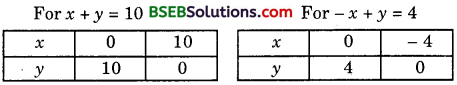Plot the points and draw the lines passing through them to represent the equations as shown.
These two lines intersect at (3, 7). So, x = 3 and y = 7 is the required solution.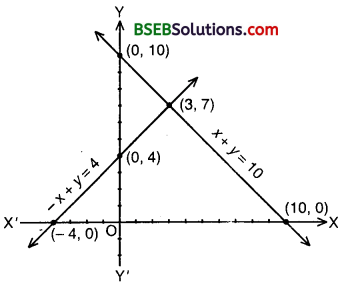Hence, the number of boys and girls are 3 and 7 respectively.

Verification:
Put x = 3 and y = 7 in (1) and (2), we find that both the equations are satisfied.

2. Let us denote the cost of one pencil by Rs x and one pen by Rs y. Then, the equations, formed are
5x + 7y = 50 ……………….. (1)
and 7x + 5y = 46 ………………… (2)
Let us draw the graphs of equations (1) and (2) by finding two solutions for each of these equations. The solutions of the equations are given in tables: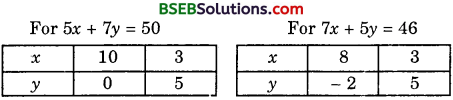Plot the points and draw the lines passing through them to represent the equations as shown.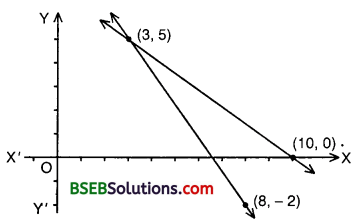These two lines intersect at (3, 5). So, x = 3 and y = 5 is the required solution.
Hence, the cost of one pencil is Rs 3 and that of one pen is Rs 5.

Verification:
Put x = 3 and y = 5 in (1) and (2), we find that both the equations are satisfied.Question 2.
On comparing the ratios $$\frac{a_{1}}{a_{2}}$$, $$\frac{b_{1}}{b_{2}}$$ and $$\frac{c_{1}}{c_{2}}$$, find out whether the lines representing the following pairs
of linear equations intersect at a point, are parallel or coincide.

1. 5x – 4y + 8 = 0; 7x + 6y – 9 = 0
2. 9x + 3y + 12 = 0; 18x + 6y + 24 = 0
3. 6x – 3y + 10 = 0; 2x – y + 9 = 0

Solution:
1. The given pair of linear equations is
5x – 4y + 8 = 0 ……………… (1)
and 7x + 6y – 9 = 0 …………….. (2)
Here, $$\frac{9}{18}$$ = $$\frac{3}{6}$$ = $$\frac{12}{24}$$ [∵ Each = $$\frac{1}{2}$$]
∴ lines (1) and (2) are coincident lines.

2. The given pair of linear equations is
9x + 3y + 12 = 0 ………………… (1)
and 18x + 6y + 24 = 0 ……………………… (2)
Here, $$\frac{9}{18}$$ = $$\frac{3}{6}$$ = $$\frac{12}{24}$$ [∵ Each = $$\frac{1}{2}$$]
∴ lines (1) and (2) are coincident lines.

3. The given pair of linear equations is
6x – 3y + 10 = 0 ……………….. (1)
and 2x – y + 9 = 0 ……………… (2)
Here, $$\frac{6}{2}$$ = $$\frac{-3}{-1}$$ ≠ $$\frac{10}{9}$$
∴ lines (1) and (2) are parallel lines.

Question 3.
On comparing the ratios $$\frac{a_{1}}{a_{2}}$$, $$\frac{b_{1}}{b_{2}}$$ and $$\frac{c_{1}}{c_{2}}$$, find out whether the following pairs of linear equations are consistent, or inconsistent.

1. 3x + 2y = 5; 2x – 3y = 7
2. 2x – 3y = 8; 4x – 6y = 9
3. $$\frac{3}{2}$$x + $$\frac{5}{3}$$y = 7; 9x – 10y = 14
4. 5x – 3y = 11; – 10x + 6y = – 22
5. $$\frac{4}{3}$$x + 2y = 8; 2x + 3y = 12

Solution:
1. 3x + 2y = 5; 2x – 3y = 7. That is, they are:
3x + 2y – 5 = 0; 2x – 3y – 7 = 0
a1 = 3, b1 = 2, c1 = – 5; a2 = 2, b2 = – 3, c2 = – 7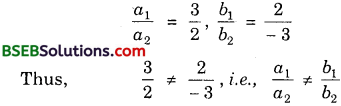∴ The pair of linear equations is consistent.

2. 2x – 3y = 8; 4x – 6y = 9. That is they are 2x – 3y – 8 = 0; 4x – 6y – 9 = 0
a1 = 2, b1 = – 3, c1 = – 8; a2 = 4, b2 = – 6, c2 = – 9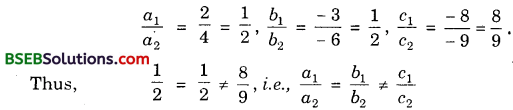∴ The pair of linear equations is consistent.

3. $$\frac{3}{2}$$x + $$\frac{5}{3}$$y = 7; 9x – 10y = 14. That is, they are $$\frac{3}{2}$$x + $$\frac{5}{3}$$y – 7 = 0 9x – 10y – 14 = 0
a1 = $$\frac{3}{2}$$, b1 = $$\frac{5}{3}$$, c1 = – 7; a2 = 9, b2 = – 10, c2 = – 14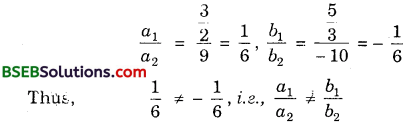∴ The pair of linear equations is consistent.

4. 5x – 3y = 11; – 10x + 6y = – 22. That is, they are:
5x – 3y – 11 = 0; – 10x + 6y + 22 = 0
a1 = 5, b1 = – 3, c1 = – 11; a2 = – 10, b2 = 6, c2 = 22∴ The pair of linear equations is consistent (and dependent).

5. $$\frac{4}{3}$$x + 2y = 8; 2x + 3y = 12. That is, they are:
$$\frac{4}{3}$$x + 2y – 8 = 0; 2x + 3y – 12 = 0
a1 = $$\frac{4}{3}$$, b1 = 2, c1 = – 8; a2 = 2, b2 = 3, c2 = – 12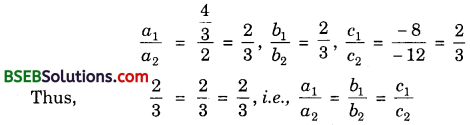∴ The pair of linear equations is consistent (and dependent).Question 4.
Which of the following pairs of linear equations are consistent? Obtain the solution in such cases graphically.

1. x + y = 5, 2x + 2y = 10
2. x – y = 8, 3x – 3y = 16
3. 2x + y – 16 = 0, 4x – 2y – 4 = 0
4. 2x – 2y – 2 = 0, 4x – 4y – 5 = 0

Solution:
1. Graph of x + y = 5 :
We have: x + y = 5 or y = 5 – x
When x = 0, y = 5; when x = 5, y = 0.
Thus, we have the following table:Plot the points A(0, 5) and B(5, 0) on the graph paper, Join A and B and extend it on both sides to obtain the graph of x + y = 5.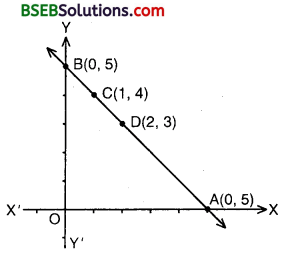Graph of 2x + 2y = 10:
We have: 2x + 2y = 10 or 2y = 10 – 2x
When x = 1, y = 5 – 1 = 4; when x = 2, y = 5 – 2 = 3
Thus, we have the following table: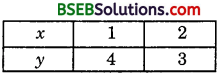Plotting the points C(1, 4) and D(2, 3) on the graph paper and drawing a line passing through these points on the same graph paper, we obtain the graph of 2x + 2y = 10. We find that C and D both lie on the graph of x + y = 5. Thus, the graphs of the two equations are coincident. Consequently, every solution of one equation is a solution of the other. Hence, the system of equations has infinitely many solutions, i.e., consistent and dependent.

2. Graph of x – y = 8:
We have: x – y = 8 or y = x – 8
When x = 0, y = – 8 when x = 8, y = 0
Thus, we have the following table: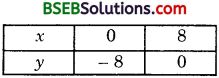Plot the points A(0, – 8) and B(8, 0) on a graph paper. Join A and B and extend it on both sides to obtain the graph of x – y = 8 as shown.
Graph of 3x – 3y = 16:
We have: 3x – 3y = 16 or 3y = 3x – 16 or y = $$\frac{3x-16}{3}$$
When x = 0, y = $$\frac{-16}{3}$$ = – 5$$\frac{1}{3}$$;
When x = $$\frac{16}{3}$$ = 5$$\frac{1}{3}$$, y = 0
Thus, we have the following table: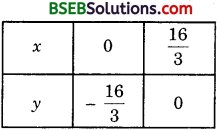Plot the points C(0, $$\frac{-16}{3}$$) and D($$\frac{16}{3}$$, 0) on the same graph paper. Join C and D and extend it on both sides to obtain the graph of 3x – 3y = 16 as shown. We find the graphs of x – y = 8 and 3x – 3y = 16 are parallel. So, the two lines have no common point. Hence, the given equations has no solution, i.e., inconsistent.3. Graph of 2x + y – 6 = 0:
We have: 2x + y – 6 = 0 or y = 6 – 2x
When x = 0, y = 6 – 0 = 6; when x = 3, y = 6 – 6 = 0
Thus, we have the following table: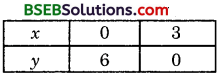Plot the points A(0, 6) and B(3, 0) on a graph paper. Join A and B and extend it on both sides to obtain the graph of 2x + y – 6 = 0 as shown.
Graph of 4x – 2y – 4 = 0:
We have: 4x – 2y – 4 = 0 or 2y = 4x – 4 or y = 2x – 2
When x = 0, y = – 2; when x – 1, y = 0
Thus, we have the following table: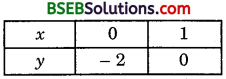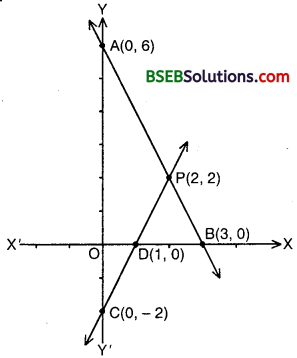Plotting the points C(0, – 2) and D(2, 0) on the same graph and drawing a line joining them as shown, we obtain the graph of 4x – 2y – 4 = 0.
Clearly, the two lines intersect at point P(2, 2).
Hence, x = 2, y = 2 is the solution of the given equations, i.e., consistent.

Verification:
Putting x = 2, y = 2 in the given equations, we find that both the equations are satisfied.

4. Graph of 2x – 2y – 2 = 0:
We have:
2x – 2y – 2 = 0 or 2y = 2x – 2 or y = x – 1
When x = 2, y = 0; when x = 0, y = – 2
Thus, we have the following table: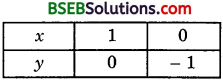Plot the points A(1, 0) and B(0, – 1) on a graph paper. Join A and B and extend it on both sides to obtain the graph of 2x – 2y – 2 = 0.
Graph of 4x – 4y – 5 = 0:
We have:
4x – 4y – 5 = 0 or 4y = 4x – 5 or y = $$\frac{4x-5}{4}$$
When x = 0, y = – $$\frac{5}{4}$$; when x = $$\frac{5}{4}$$, y = 0.
Thus, we have the following table:Plot the points C(0, $$\frac{- 5}{4}$$) and D($$\frac{5}{4}$$, 0) on the same graph paper. Join C and D and extend it on both sides to obtain the graph of 4x – 4y – 5 = 0 as shown. We find the graphs of these equations are parallel lines. So, the two lines have no common point. Hence, the given system of equations has no solution, i.e., inconsistent.Question 5.
Half the perimeter of a rectangular garden, whose length is 4 m more than its width, is 36 m. Find the dimensions of the garden.
Solution:
Let the length of the garden be x m and its width be y m.
Then, perimeter = 2( Length + Width)
= 2(x + y)
Therefore, half perimeter = (x + y)
But it is given as 36
∴ (x + y) = 36 ………………. (1)
Also, x = y + 4 i.e; x – y = 4 ………………… (2)
For finding the solution of (1) and (2) graphically, we form the following tables: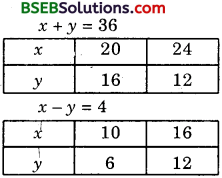Draw the graphs by joining points (20, 16) and (24, 12) and points (10, 6) and (16, 12). The two lines intersect at point (20, 16) as shown in the figure.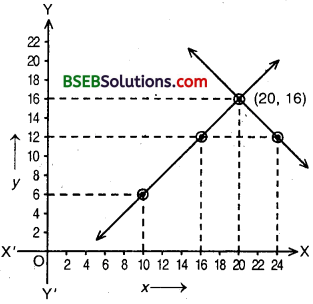Hence, Length = 20 m and width = 16 m.

Question 6.
Given the linear equation 2x + 3y – 8 = 0, write another linear equation in two variables such that the geometrical representation of the pair so formed is:

1. intersecting lines
2. parallel lines
3. coincident lines

Solution:
Given linear equation is 2x + 3y – 8 = 0 ……………… (1)
1. For intersecting lines, we know that
$$\frac{a_{1}}{a_{2}} \neq \frac{b_{1}}{b_{2}}$$
Any intersecting line may be taken as
5x + 2y – 9 – 0

2. For parallel lines, $$\frac{a_{1}}{a_{2}}$$ = $$\frac{b_{1}}{b_{2}}$$ ≠ $$\frac{c_{1}}{c_{2}}$$
Any line parallel to (1) may be taken as
4x + 6y – 3 = 0

3. For coincident lines, $$\frac{a_{1}}{a_{2}}$$ = $$\frac{b_{1}}{b_{2}}$$ = $$\frac{c_{1}}{c_{2}}$$
Any line coincident to (1) may be taken as
6x + 9x – 24 = 0
Note: Answers can differ from person to person.Question 7.
Draw the graphs of the equations x – y + 1 = 0 and 3x + 2y – 12 = 0. Determine the coordinates of the vertices of the triangle formed by these lines and the x – axis, and shade the triangular region.
Solution:
For the graph of x – y + 1 = 0:
We have:
x – y + 1 = 0 or y = x + 1
When x = 0, y = 1; when x = – 1, y’= 0.
Thus, we have the following table:Plot the points A(0, 1) and B(- 1, 0) on a graph paper. Join A and B and extend it on both sides to obtain the graph of x – y + 1 = 0.For the graph of 3x + 2y – 12 = 0:
We have:
3x + 2y – 12 = 0 or 2y = 12 – 3x or y = $$\frac{12-3x}{2}$$
When x = 4, y = $$\frac{12-12}{2}$$ = 0
When x = 0, y = $$\frac{12}{2}$$ = 6
Thus, we have the following table: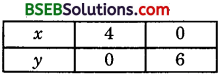Plot the points C(4, 0) and D(0, 6) on the same graph paper and draw a line passing through these two points to obtain the graph of equation 3x + 2y – 12 = 0. Clearly, we obtain a ∆ PBC formed by the given lines and the x-axis. The co-ordinates of the vertices are P(2, 3), B(- 1, 0) and C(4, 0). Triangular region is shaded as shown.